Next: H Arnoldi Algorithm Up: Dissertation Christoph Wasshuber Previous: F Second Order Co

The (p,q) Padé approximant to the matrix exponential eX is, by definition, the unique (p,q) rational function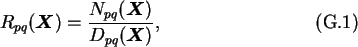which matches the Taylor series expansion of eX through terms to the power p+q. Its coefficients are therefore determined by solving the algebraic equations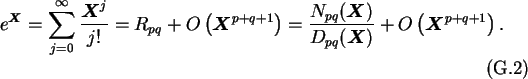The result is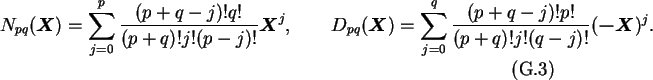Choosing p=q one obtains the diagonal Padé approximation. This choice is to prefer, because it yields a higher order approximation with the same amount of computation.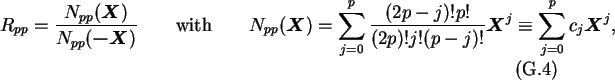where the coefficients cj can be conveniently constructed by means of the recursion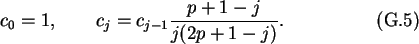The computation of the polynomials are best done with a Horner scheme. C. Moler and C. Van Loan  showed that if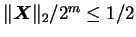, then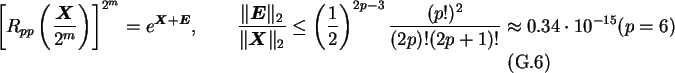Christoph Wasshuber